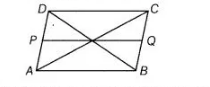# The diagonals of a parallelogram ABCD`
Question:

The diagonals of a parallelogram ABCD intersect at a point O. Through O, a line is drawn to intersect AD at P and BC at Q. Show that PQ divides the

parallelogram into two parts of equal area.

Solution:

Given in a parallelogram $A B C D$, diagonals interect at $O$ and draw a line $P Q$, which intersects $A D$ and $B C$.

To prove $P Q$ divides the parallelogram $A B C D$ into two parts of equal area.

i.e. ar $(A B Q P)=\operatorname{ar}(C D P Q)$Proof We know that, diagonals of a parallelogram bisect each other.

$\therefore \quad . O A=O C$ and $O B=O D$ ......(i)

In $\triangle A O B$ and $\triangle C O D$,

$O A=O C$

$O B=O D$ [from Eq. (i)]

and  $\angle A O B=\angle C O D$  [vertically opposite angles]

$\therefore$ $\triangle A O B \cong \triangle C O D$ [by SAS congruence rule]

Then, $\operatorname{ar}(\Delta A O B)=\operatorname{ar}(\Delta C O D)$ .....(ii)

[since, congruent figures have equal area]

Now, in $\triangle A O P$ and $\triangle C O Q$.

$\angle P A O=\angle O C Q \quad$ [alternate interior angles]

$O A=O C \quad \quad$ [from Eq. (i)]

and $\quad \angle A O P=\angle C O Q \quad$ [vertically opposite angles]

$\therefore \quad \Delta A O P \cong \Delta C O Q \quad$ [by ASA congruence rule]

$\therefore \quad \operatorname{ar}(\Delta A O P)=\operatorname{ar}(\Delta C O Q)$ ... (iii)

[since, congruent fiqures have equal area]

Similarly, $\operatorname{ar}(\Delta P O D)=\operatorname{ar}(\triangle B O Q)$

Now, $\quad \operatorname{ar}(A B Q P)=\operatorname{ar}(\Delta C O Q)+\operatorname{ar}(\Delta C O D)+\operatorname{ar}(\triangle P O D)$

$=\operatorname{ar}(\Delta A O P)+\operatorname{ar}(\Delta A O B)+\operatorname{ar}(\Delta B O Q)$

[from Eqs. (ii), (iii) and (iv)]

$\Rightarrow \quad \operatorname{ar}(A B Q P)=\operatorname{ar}(C D P Q) \quad$ Hence proved.